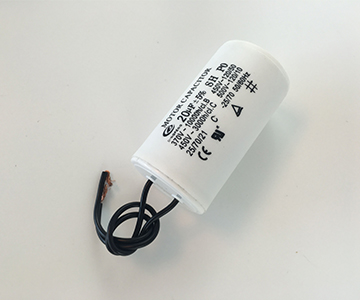News Group
Dingfeng Capacitor--Capacitor Values – Prefixes, Standards and Calculations
Issue Time:2019-04-28
Capacitor Values – Prefixes, Standards and CalculationsThere are some standard capacitor values that have developed over time. To find the value you need for your circuit you need to know how to deal with prefixes. And you need to know how to calculate capacitor values.

If you have looked for capacitors, you have probably seen many different letters and weird values. Like 0.47 \mu F or 22 pF. It is a bit confusing, but it’s easy to learn what it means

In this article you will learn the most standard capacitor values, the prefixes used and how to calculate a capacitor value for your circuit.

The Prefixes
Capacitor values are given in Farad. The symbol used is F. It’s named after the English physicist Michael Faraday.

But 1 Farad is pretty big. So capacitor values are usually given with a prefix. Often you are going to work with capacitors values in pico-farads to micro-farads.

To make this simpler to deal with, I’m going to show you how the prefixes work.

A prefix is something you put in front of the farad symbol (F). It tells you what you have to multiply the number with. For example 1 pF means 1 F multiplied with the prefix p which is 10^(-12). It can also be written 0.000000000001

Here is a table with the most common prefixes for capacitors:

How Do You Choose Capacitor Values?

You choose a capacitor value by using the RC time constant:

\tau = R * C

This constant gives you the time it takes for a voltage in an RC circuit to go from 0% to 63% of its full value.

You can use this time constant to calculate the cutoff frequency in a filter, or just how long a delay will be in a blinking light circuit.

Calculating Capacitor Value For A Circuit

Low-pass filter using a capacitor and a resistor

Low-pass filter using a capacitor and a resistor

Lets say we want to make a low pass filter with a cutoff frequency of 15 kHz. The formula for calculating cutoff frequency is:

f_{c} = \frac{1}{2 \pi RC}

and by switching it around we can calculate for C:

C = \frac{1}{2 \pi R f_{c}}

Now, lets choose to use a 1k resistor. This gives us:

C = \frac{1}{2 * 3.14 * 1000 \Omega * 15000 Hz} = 0.000000011 = 11 nF

So to get a cutoff frequency of 15 kHz, we need a 1k resistor and a 11 nF capacitor.

Different Types of Capacitors

To make everything more confusing, capacitors come in many different types. I have written a simple guide on how to choose a capacitor type that you should check out.

There is no need to memorize these things. Just bookmark this page and youll have easy access to it later on. When you have used the prefixes and the formula enough times, you will automatically remember them.